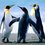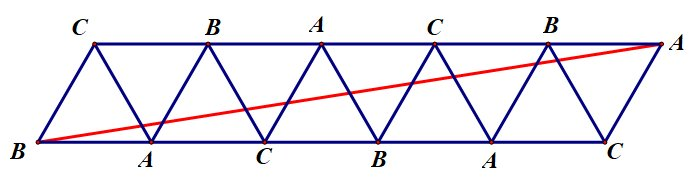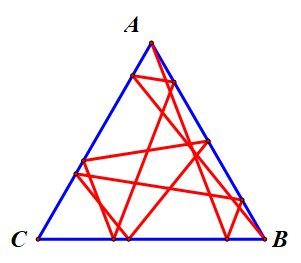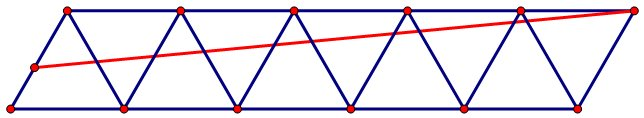# Super ball

A friction-less board has the shape of an equilateral triangle of side length $1$ meter with bouncing walls along the sides. A tiny super bouncy ball is fired from vertex $A$ towards the side $BC$. The ball bounces off the walls of the board nine times before it hits a vertex for the first time. The bounces are such that the angle of incidence equals the angle of reflection. The distance travelled by the ball in meters is ?Note by Kudo Shinichi
1 year, 11 months ago

This discussion board is a place to discuss our Daily Challenges and the math and science related to those challenges. Explanations are more than just a solution — they should explain the steps and thinking strategies that you used to obtain the solution. Comments should further the discussion of math and science.

When posting on Brilliant:

• Use the emojis to react to an explanation, whether you're congratulating a job well done , or just really confused .
• Ask specific questions about the challenge or the steps in somebody's explanation. Well-posed questions can add a lot to the discussion, but posting "I don't understand!" doesn't help anyone.
• Try to contribute something new to the discussion, whether it is an extension, generalization or other idea related to the challenge.

MarkdownAppears as
*italics* or _italics_ italics
**bold** or __bold__ bold
- bulleted- list
• bulleted
• list
1. numbered2. list
1. numbered
2. list
Note: you must add a full line of space before and after lists for them to show up correctly
paragraph 1paragraph 2

paragraph 1

paragraph 2

[example link](https://brilliant.org)example link
> This is a quote
This is a quote
    # I indented these lines
# 4 spaces, and now they show
# up as a code block.

print "hello world"
# I indented these lines
# 4 spaces, and now they show
# up as a code block.

print "hello world"
MathAppears as
Remember to wrap math in $$ ... $$ or $ ... $ to ensure proper formatting.
2 \times 3 $2 \times 3$
2^{34} $2^{34}$
a_{i-1} $a_{i-1}$
\frac{2}{3} $\frac{2}{3}$
\sqrt{2} $\sqrt{2}$
\sum_{i=1}^3 $\sum_{i=1}^3$
\sin \theta $\sin \theta$
\boxed{123} $\boxed{123}$

Sort by:

Consider an array of triangles as shown below, with vertices labelled as shown. The red straight line travels from a vertex A to a vertex B, crossing $9$ edges in the process.Now fold up the red line, reflecting each portion of the line in the triangle edge it crosses, and we obtain the following track of a ball from vertex A to vertex B, which bounces perfectly off 9 walls.The original red length moves $5\tfrac12$ m horizontally and $\tfrac12\sqrt{3}$ m vertically, and hence is $\sqrt{31}$ m long. Thus the ball's path is $\sqrt{31}$ m long.

- 1 year, 11 months ago

Amazing solution @Mark Hennings Sir , btw if ball was thrown from a point in the line AB , except the vertices , can then also the ball will land on a vertice after nine times striking the walls? Is it possible ?

- 1 year, 11 months ago

Try playing with a triangular grid and drawing lines. You should be able to convince yourself that you can get from anywhere on an edge to some vertex after any required number of bounces..- 1 year, 11 months ago

Oh wow , such a beauty of this problem, btw @Mark Hennings Sir , how u think of the idea of making equilateral triangles copies by reflecting many times ?

- 1 year, 11 months ago

Also @Mark Hennings sir is it possible for ball to land at same vertex , from where it started ?

- 1 year, 11 months ago

Fire the ball from one vertex towards the midpoint of the other side, and it will bounce straight back to the vertex it started from. There are other less trivial solutions... Try to find them yourself. I can get from a vertex back to itself after seven bounces, for example.

Coping with bounces by this process of reflection is quite standard. You might have seen it before with a rectangular table, and bouncing a pool ball...

- 1 year, 11 months ago

Got it now @Mark Hennings sir thx a lot.

- 1 year, 11 months ago

You're welcome

- 1 year, 11 months ago

@Steven Chase sir @Mark Hennings sir pls see this problem

- 1 year, 11 months ago